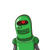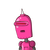# if (0,0),(2,0),(0,3),are vertices of a triangle then find its area​

if (0,0),(2,0),(0,3),are vertices of a triangle then find its area​

### 2 thoughts on “if (0,0),(2,0),(0,3),are vertices of a triangle then find its area​”

1.we have area formula of triangle,

Δ = 1/2 [ x₁ ( y₂ – y₃ ) + x₂ ( y₃ – y₁ ) + x₃ ( y₁ – y₂ ) ]

(x₁ , y₁) = ( 0 , 0 )  , ( x₂ , y₂ ) = ( 2 , 0 ) , ( x₃ , y₃ ) = ( 0 , 3 )

Δ = 1/2 [ 0 ( 0 – 3 ) + 2 ( 3 – 0 ) + 0 ( 0 – 3 )

Δ = 1/2 * 6

Δ = 3 unit² (Ans.)

2.